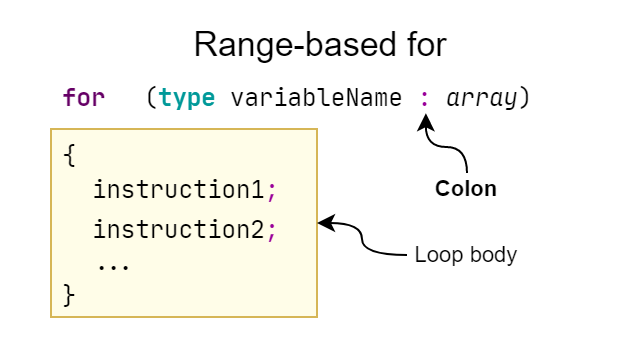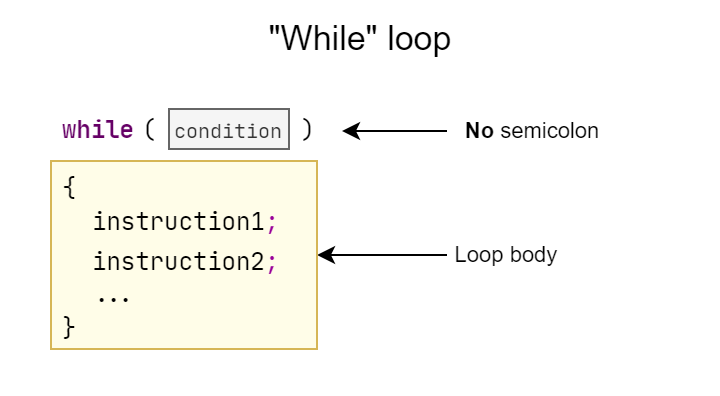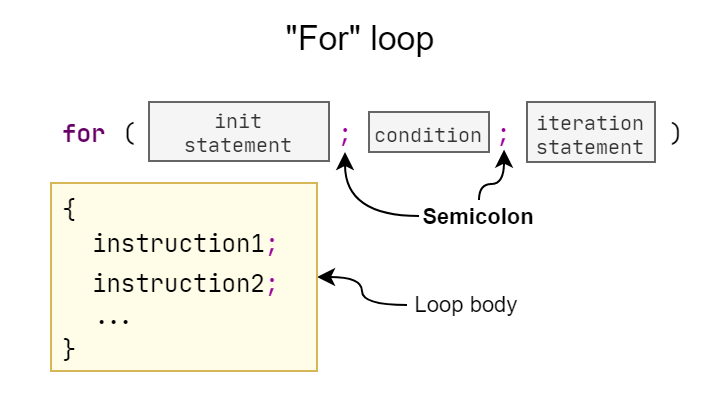caution

# Loops

In this lesson, we'll make the program execute a given code multiple times, that is, we'll use a loop.

## Motivation​

Loops have many uses, here are a few of them:

• 👾 adding, for example, 10 new opponents to the level in the game
• 🖥 displaying each element in an array
• ➗ multiple calculations (e.g. counting factorials, fibonacci sequence)

In the lesson about vectors, we already showed one loop that displayed all the elements of an array:

🔹 Print each number from an array
``for (int n : numbers){    std::cout << n << ' ';}``

This is the simplest version of a loop in C++. You will learn more about their types in the following sections.

## Loop types​

In C++, we have the following loops:

• `for`
• range-based version
• basic version
• `while`
• `do ... while`

The most common used are `for` and `while`, and we'll cover them in this lesson. If you want to read about the `do ... while` loop, see the article: `do ... while` loop.

Iteration (definition)

Iteration - a single loop body execution.

## Explaination​

### Range-based `for`​

This type of loop is most commonly used for working with arrays, although it can also be used in other ways.Scheme

In the example shown in the Motivation section, there is a range-based for loop, which is a version of the `for` loop for the so-called ranges. An array as understood in C++ is also a range, so feel free to use it.

The simplest example:

🔹 Print each number from an array
``std::vector<int> numbers = { 13, 42, -1, 0, -3, -5 };for (int n : numbers){    std::cout << n << ' ';}``

This loop sequentially traverses each element of the array `numbers` and writes it to the variable `n`. The block of code enclosed in the curly braces is then executed. In this case it is a simple printing of the number.

Colon

Note that the variable name `n` is followed by a colon (`:`), not a semicolon (`;`)! We do not use any equal sign (`=`) in this notation, because the value of each element in turn will be automatically assigned to `n`.

## `while` loop​Scheme

We are now intentionally moving into the `while` loop, rather than the basic `for`, as this will make the explanation easier. The while loop executes its body as long as the condition is met:

🔹 Print numbers from 0 to 3
``int number = 0;while (number <= 3){    std::cout << number << ' ';    number++;}``
Result
``0 1 2 3``

The condition will be checked before each loop execution and as long as it is satisfied, i.e. as long as `number` is less than or equal to `3` in this case, the body will be executed.

• printing the `number`
• increasing `number` by 1

After the last loop execution, the value of `number` will be equal to `4`, so the condition will not be satisfied, causing the loop to terminate and the computer to proceed with the next instructions.

### `for` loop​Schema

This loop is a simplification of a certain very frequently repeated pattern, and it is typically used to step through some range (such as numeric range).

🔹 Display numbers from 0 to 9
``for (int i = 0; i < 10; i++){    std::cout << i << ' ';}``

The above loop displays the numbers from 0 to 9. The round bracket at `for` consists of three parts, separated by semicolons:

FragmentDescription
`int i = 0`initial instruction (usually the creation of a variable)
`i < 10`condition
`i++`iteration expression

When a program starts executing a `for` loop, it executes the initial statement once - in our case creates a variable and gives it the value `0`. The program then:

1. checks the condition
• unfulfilled: leave the loop
• fulfilled: go to the point 2
2. executes the loop body
3. performs an iteration expression and goes to pt. 1

The above `for` loop is equivalent to:

``int i = 0;while (i < 10){    std::cout << i << ' ';    i++;}``

#### Iterating through arrays​

The `for` loop is very often used to iterate through arrays, in situations where we either need access to the iteration number or we do not want to iterate over the entire range.

🔹 Iterating over an entire array
``std::vector<int> numbers = {10, 13, 15, 18, 60};for (int i = 0; i < numbers.size(); i++){    std::cout << "numbers[" << i << "]: " << numbers[i] << '}}``
🔹 Iterating over half of an array
``std::vector<int> numbers = {10, 13, 15, 18, 60};for (int i = 0; i < numbers.size() / 2; i++){    std::cout << "numbers[" << i << "]: " << numbers[i] << '}}``

#### Empty parenthesis​

The code given in the `for` loop parentheses is optional. The semicolons are required.

🔹 For loop with empty parentheses
``for ( ; ; ){    // code}``

The above will make the `for` loop execute infinitely (due to the empty condition), unless we manually break it...

## Stopping a loop​

We can stop the loop at any point using the `break` statement:

``for (int i = 0; i < 10; i++){    if (i == 5)        break;    std::cout << i << ' ';}``

This loop will display numbers from 0 to 4, because at `i` equal to `5` the execution of the loop will be aborted. We can break the `while` loop in the same way.

## Skipping a single iteration​

To skip further execution of the current loop iteration we use the `continue` statement:

``for (int i = 0; i < 10; i++){    if (i == 5)        continue;    std::cout << i << ' ';}``

The loop will display the numbers from `0` to `9`, excluding the number `5`, because before it executes the display instruction (`std::cout`) the program will jump to the next iteration.

For loop and continue

Note that using `continue` in a `for` loop does not omit the iteration expression (see diagram above).

## Examples​

info

This section requires improvement. You can help by editing this doc page.

## Potential errors​

info

This section requires improvement. You can help by editing this doc page.

info

This section requires improvement. You can help by editing this doc page.

caution

# Loops

In this lesson, we'll make the program execute a given code multiple times, that is, we'll use a loop.

## Motivation​

Loops have many uses, here are a few of them:

• 👾 adding, for example, 10 new opponents to the level in the game
• 🖥 displaying each element in an array
• ➗ multiple calculations (e.g. counting factorials, fibonacci sequence)

In the lesson about vectors, we already showed one loop that displayed all the elements of an array:

🔹 Print each number from an array
``for (int n : numbers){    std::cout << n << ' ';}``

This is the simplest version of a loop in C++. You will learn more about their types in the following sections.

## Loop types​

In C++, we have the following loops:

• `for`
• range-based version
• basic version
• `while`
• `do ... while`

The most common used are `for` and `while`, and we'll cover them in this lesson. If you want to read about the `do ... while` loop, see the article: `do ... while` loop.

Iteration (definition)

Iteration - a single loop body execution.

## Explaination​

### Range-based `for`​

This type of loop is most commonly used for working with arrays, although it can also be used in other ways.Scheme

In the example shown in the Motivation section, there is a range-based for loop, which is a version of the `for` loop for the so-called ranges. An array as understood in C++ is also a range, so feel free to use it.

The simplest example:

🔹 Print each number from an array
``std::vector<int> numbers = { 13, 42, -1, 0, -3, -5 };for (int n : numbers){    std::cout << n << ' ';}``

This loop sequentially traverses each element of the array `numbers` and writes it to the variable `n`. The block of code enclosed in the curly braces is then executed. In this case it is a simple printing of the number.

Colon

Note that the variable name `n` is followed by a colon (`:`), not a semicolon (`;`)! We do not use any equal sign (`=`) in this notation, because the value of each element in turn will be automatically assigned to `n`.

## `while` loop​Scheme

We are now intentionally moving into the `while` loop, rather than the basic `for`, as this will make the explanation easier. The while loop executes its body as long as the condition is met:

🔹 Print numbers from 0 to 3
``int number = 0;while (number <= 3){    std::cout << number << ' ';    number++;}``
Result
``0 1 2 3``

The condition will be checked before each loop execution and as long as it is satisfied, i.e. as long as `number` is less than or equal to `3` in this case, the body will be executed.

• printing the `number`
• increasing `number` by 1

After the last loop execution, the value of `number` will be equal to `4`, so the condition will not be satisfied, causing the loop to terminate and the computer to proceed with the next instructions.

### `for` loop​Schema

This loop is a simplification of a certain very frequently repeated pattern, and it is typically used to step through some range (such as numeric range).

🔹 Display numbers from 0 to 9
``for (int i = 0; i < 10; i++){    std::cout << i << ' ';}``

The above loop displays the numbers from 0 to 9. The round bracket at `for` consists of three parts, separated by semicolons:

FragmentDescription
`int i = 0`initial instruction (usually the creation of a variable)
`i < 10`condition
`i++`iteration expression

When a program starts executing a `for` loop, it executes the initial statement once - in our case creates a variable and gives it the value `0`. The program then:

1. checks the condition
• unfulfilled: leave the loop
• fulfilled: go to the point 2
2. executes the loop body
3. performs an iteration expression and goes to pt. 1

The above `for` loop is equivalent to:

``int i = 0;while (i < 10){    std::cout << i << ' ';    i++;}``

#### Iterating through arrays​

The `for` loop is very often used to iterate through arrays, in situations where we either need access to the iteration number or we do not want to iterate over the entire range.

🔹 Iterating over an entire array
``std::vector<int> numbers = {10, 13, 15, 18, 60};for (int i = 0; i < numbers.size(); i++){    std::cout << "numbers[" << i << "]: " << numbers[i] << '}}``
🔹 Iterating over half of an array
``std::vector<int> numbers = {10, 13, 15, 18, 60};for (int i = 0; i < numbers.size() / 2; i++){    std::cout << "numbers[" << i << "]: " << numbers[i] << '}}``

#### Empty parenthesis​

The code given in the `for` loop parentheses is optional. The semicolons are required.

🔹 For loop with empty parentheses
``for ( ; ; ){    // code}``

The above will make the `for` loop execute infinitely (due to the empty condition), unless we manually break it...

## Stopping a loop​

We can stop the loop at any point using the `break` statement:

``for (int i = 0; i < 10; i++){    if (i == 5)        break;    std::cout << i << ' ';}``

This loop will display numbers from 0 to 4, because at `i` equal to `5` the execution of the loop will be aborted. We can break the `while` loop in the same way.

## Skipping a single iteration​

To skip further execution of the current loop iteration we use the `continue` statement:

``for (int i = 0; i < 10; i++){    if (i == 5)        continue;    std::cout << i << ' ';}``

The loop will display the numbers from `0` to `9`, excluding the number `5`, because before it executes the display instruction (`std::cout`) the program will jump to the next iteration.

For loop and continue

Note that using `continue` in a `for` loop does not omit the iteration expression (see diagram above).

## Examples​

info

This section requires improvement. You can help by editing this doc page.

## Potential errors​

info

This section requires improvement. You can help by editing this doc page.